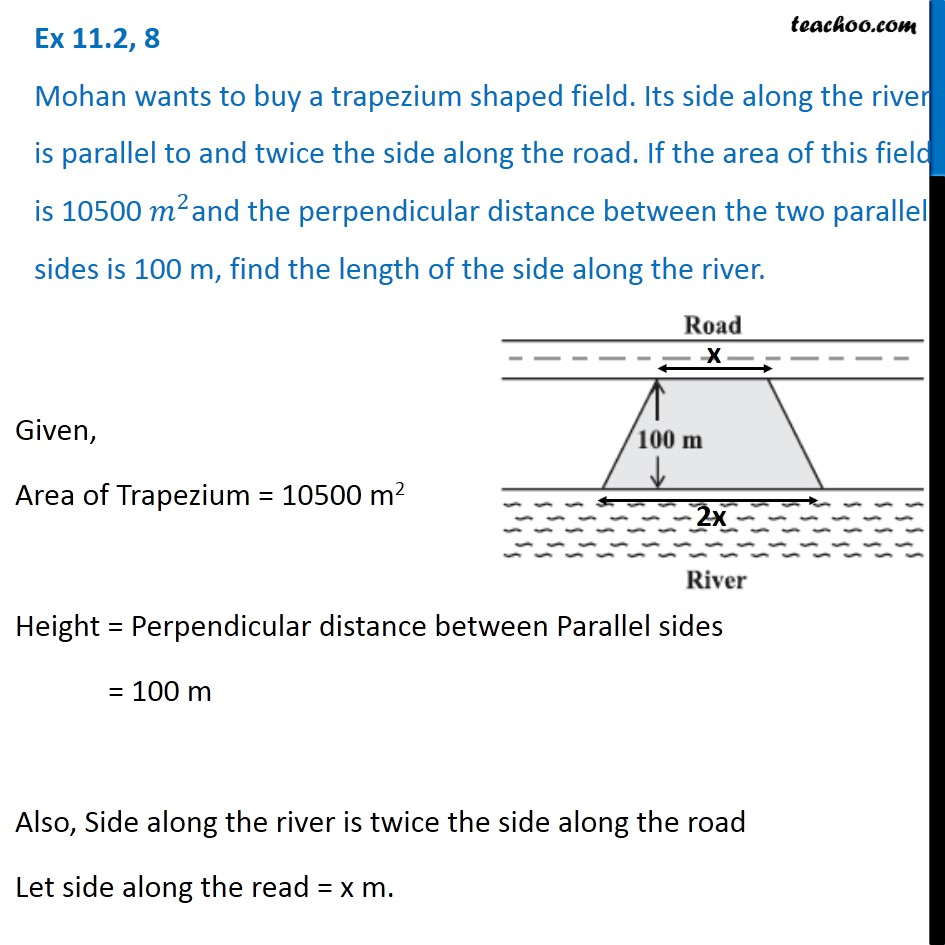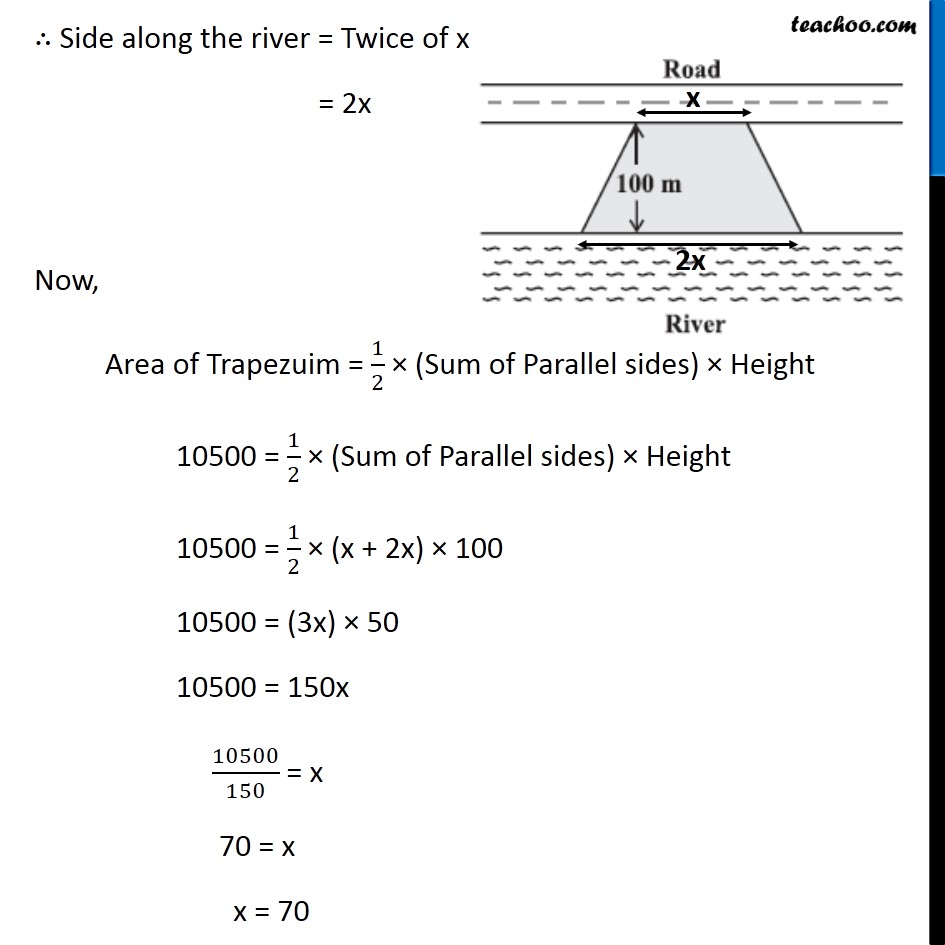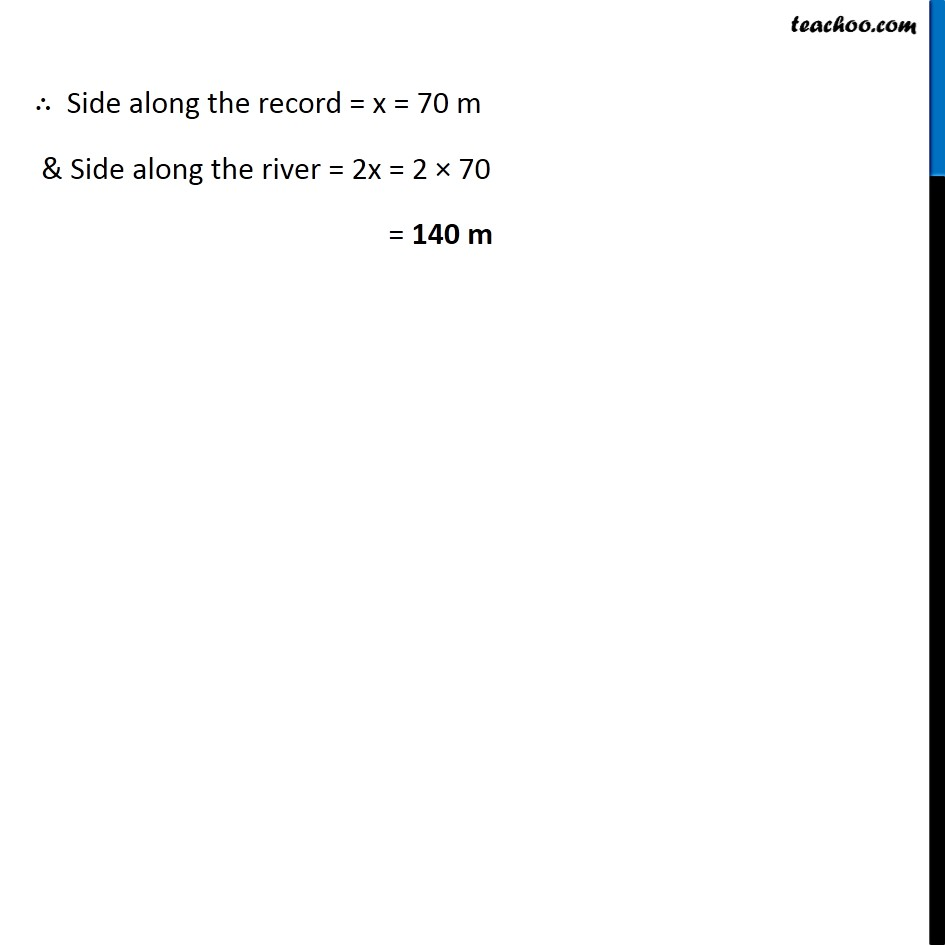Ex 11.2

Chapter 11 Class 8 Mensuration
Serial order wiseGet live Maths 1-on-1 Classs - Class 6 to 12

### Transcript

Ex 11.2, 8 Mohan wants to buy a trapezium shaped field. Its side along the river is parallel to and twice the side along the road. If the area of this field is 10500 𝑚^2and the perpendicular distance between the two parallel sides is 100 m, find the length of the side along the river. Given, Area of Trapezium = 10500 m2 Height = Perpendicular distance between Parallel sides = 100 m Also, Side along the river is twice the side along the road Let side along the read = x m. ∴ Side along the river = Twice of x = 2x Now, Area of Trapezuim = 1/2 × (Sum of Parallel sides) × Height 10500 = 1/2 × (Sum of Parallel sides) × Height 10500 = 1/2 × (x + 2x) × 100 10500 = (3x) × 50 10500 = 150x 10500/150 = x 70 = x x = 70 ∴ Side along the record = x = 70 m & Side along the river = 2x = 2 × 70 = 140 m